Study Guide

# Forces and Motion - Newton's Three Laws of Motion

## Newton's Three Laws of Motion

Now that’s we’ve mastered motion and vectors to at least a moderate degree, we’re prepared to study forces, another vector quantity related to motion. And how could we possibly study forces without introducing Sir Isaac Newton?

### Newton’s Laws of Motion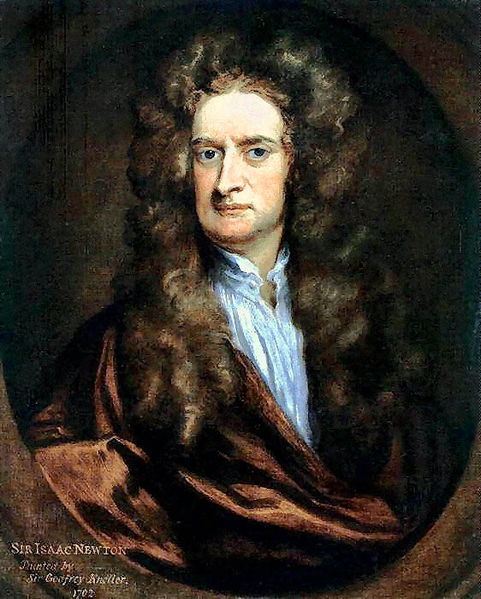Newton, otherwise known as “The Guy in the Fancy Wig”Source

In reviewing the work of Copernicus, Galileo, and Kepler, Isaac Newton connected their dots and came up with three laws of motion, first published in 1687, for which we are forever in his debt. We thank him less for developing calculus along the way, too.

### The First Law of Motion

Newton’s First Law of Motion says that an object at rest tends to stay at rest, while an object in motion tends to stay in motion. And by “motion,” we mean constant motion: an unchanging velocity. We caught a glimpse of this in the horizontal movement of a projectile. Without outside influences (forces) the motion doesn’t change by its very nature.

This law is frequently referred to as the law of inertia, where inertia is a property of mass, which in turn is a property of how much of a substance there is. Inertial mass is the measure of an object’s resistance to acceleration, the resistance to a change in motion.

We may or may not have run into this idea in our other lives. In literature, previous science classes, or by personal experience.

The more mass something has, the harder it is to get it moving or stop it once it’s whizzing through the universe. That’s the whole philosophy behind what charging linebackers do. Or stationary ones, for that matter.

We could also think of the First Law as the Couch Potato Law. If we’re vegetating on a couch, perhaps eating potato chips, we won’t be inclined to move at all. Except that it’s also true for couch potatoes moving at constant velocity, this analogy is great.

Practice time. Tweetie ends up losing 0.1 g of fat while flying his square loop so many times. Is he now less inclined or more inclined to resist change in motion? Let’s pay attention to the wording here. The question asks about Tweetie’s inclination to resist change. We now know something heavier resists change more strongly. Tweetie is (marginally) lighter after his laps, so he will be (marginally) less inclined to resist change. His inertia is (marginally) smaller.

To sum up the First Law of Motion: if an object were free to just exist outside of any gravity or electric or magnetic fields or friction or air resistance, it would either continue to be at rest or move forever in a straight line at a constant velocity.

Without trying to get too far ahead of ourselves, let’s just verify that we do indeed observe objects at rest or in constant motion in our world, but if and only if the forces on them are balanced.

### The Second Law of Motion

The converse of the First Law would say that anything that causes an object’s motion—or lack thereof—to change must exert a force on that object, because otherwise it’d continue as it was. In other words, an external force will change the object’s velocity, producing acceleration of an inertial, change-resistant object.

Obviously, we see it all the time on Earth. We don’t fly off the surface of the planet when we start running, or throw balls into outer space. We live on the surface of a planet with a constant acceleration of gravity that’s constantly pulling us toward its center with the force of gravity, which stems from its massive mass in comparison with our own.

It’s no wonder Sir Isaac Newton legendarily discovered his famous laws of motion while napping peacefully under an apple tree. The story goes that an apple fell on his head, which prompted Newton to jump to his feet, and shout Eurêka! as he ran down the street naked.

Hmm. Wait, that might’ve been another guy. Yes, someone else. Newton gracefully remained on the ground, probably ate the apple, and ultimately reasoned out what made the apple fall to the ground. He might’ve even conjured up an image similar to the one below.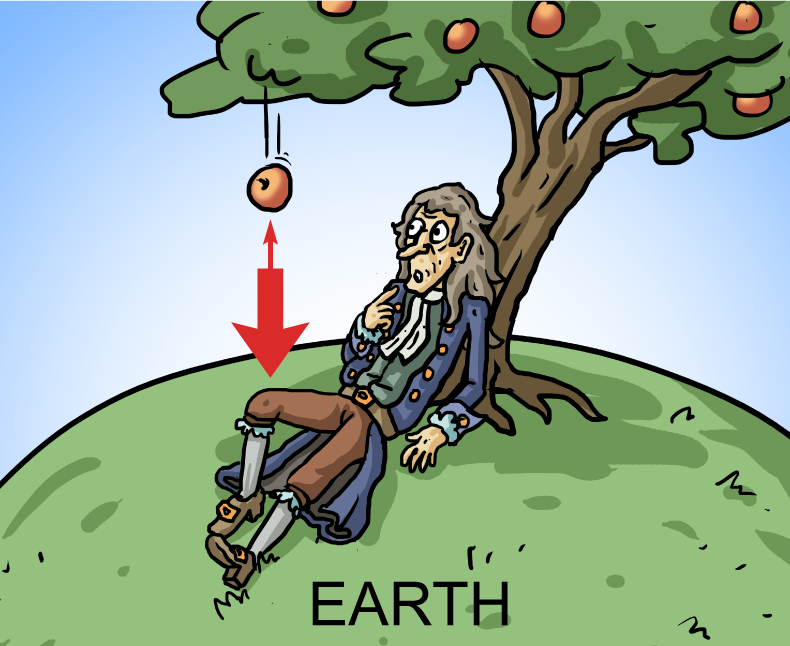The apple, beginning from a state of rest, accelerated without any visible interference. The apple, an object with mass, accelerated from the force of gravity.

This leads us to Newton’s Second Law of Motion: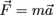.

Yes, the Second Law is math. So?

Right away, we notice that force is a vector, not unlike acceleration, and since math is math and there’s one vector on each side of the equation, the force must point in the same direction as the corresponding acceleration. And vice versa.

Force has units of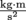from the mass times acceleration. Since that’s awkward, they’ve been appropriately renamed as Newton (N). After the man, not the cookie. If we use grams or inches or anything but SI units within the mass and acceleration, we don’t get Newtons out, but the Newton is the SI unit so let’s stick to it, shall we?

Math time: an object has a mass of 1 kg and travels at a velocity of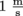. How much force is needed to double its velocity in 1 second? Uhh…what? Let’s start with identifying what we know.
We know F = ma, and that the acceleration a is given by the change in velocity over time, Δt = 1 s, with vf = 2vi, or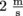. Therefore,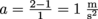, which means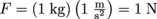for a 1 kg object.

Since practice makes perfect and there’s no time like the present, let’s try another example.

If a 2 kg sliding book covers a distance of d = 1 m in time t = 2 seconds at a steady speed, then slows before coming to a complete halt 1 second later, what amount of force acted upon it to slow it down?

Ooh, multistep question; we like it. To find the speed (or velocity, but we weren’t given a direction to account for), we get to pull out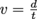, which means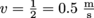. This is its initial velocity, as far as acceleration is concerned. Its final velocity is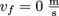, the mathematical definition of “a halt”.

To find the acceleration, we need both velocities, as well as the time between the two velocities, which is 1 second. Note that it’s not the 2 seconds from the time of constant velocity. Then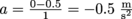. Lastly, F = ma, so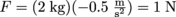. The negative sign indicates that both the acceleration and force oppose the direction of velocity, which makes sense because the object slowed down.

We could even use Newton’s Second Law to find the magnitudes of forces that balance each other, such that the sum of forces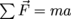. In the case of balanced forces, then there’s no net force or acceleration, and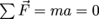.

Chances are we’re all sitting down somewhere right now. The forces on each of us in this state are the force of gravity, the inescapable (if we’re on Earth), and the force from the surface of whatever we’re sitting on holding us up. If there weren’t a force holding us up, we’d accelerate to the center of the earth.

The surface or contact force is called the normal force. “Normal” in mathematical language means “perpendicular”. The normal force pushes perpendicular to the surface. Assuming that our chairs or couches or floors or whatever we’re sitting on are level horizontally, the normal force points upward, exactly counter to the force of gravity.

If the force from gravity is 700 N each, then we’d say that Fg = 700 N, and therefore the normal force FN = 700 N, too, because the forces are balanced.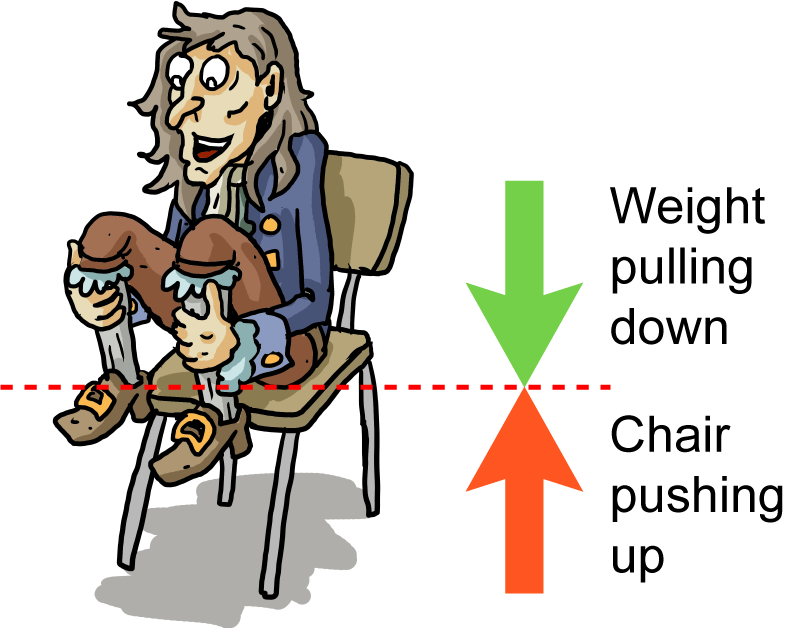Above is a force diagram for our sitting selves, otherwise known as a free-body diagram. It shows the forces as vectors acting on an object. For balanced forces, the arrows should be the same length, pointing in opposite direction. The arrows are conventionally drawn from the center of the object outward.

Next time Mom wants us to get up and help in the kitchen, we can truthfully tell her that we can’t because we’re studying balanced forces right now. By the way, balanced forces lead to an object being in equilibrium, with no acceleration. Note that an object can be in equilibrium at a constant velocity because of Newton’s First Law of Motion. Darn it, we can “study” balanced forces while walking at a steady rate to the kitchen….don’t tell Mom.

### Newton’s Third Law of Motion

To put it bluntly, Newton’s Third Law of Motion says that every action has an equal and opposite reaction, and by “action,” we mean “force”. Just to be clear. To be even more clear, FAB = -FBA, which means that the force on A from B is equal and opposite to the force on B from A.

This action-reaction idea is also popular in philosophy. Newton was really on to something with this Third Law thing.

Warning: the force of the chair pushing upward on our “seats” is not the reaction force to the force of gravity. The reaction force to the force of gravity the Earth puts on us, is the force of gravity we put on the Earth. The reaction force to the normal force of the chair pushing us up is the force of us pushing on the chair.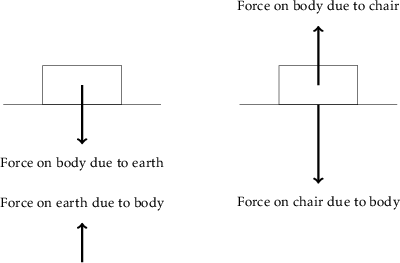Clear as mud? Newton’s Third Law is confusing like that. Let’s look at a force diagram again, err, free-body diagram. While it’s certainly true that if we put reaction forces on a force diagram, then all forces would seem balanced, but the reaction forces have no business belonging on a force diagram.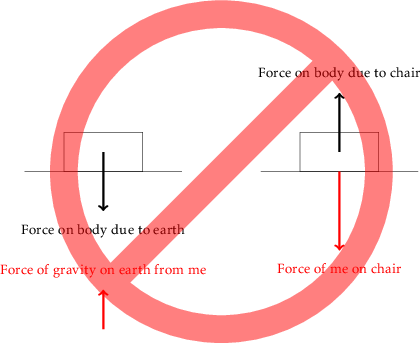The only forces on an object which belong on its free-body diagram are external forces causing acceleration on an object. If we pushed on the desk, we don’t include that push on our own FBD (free-body diagram). Rather, we include the force of the desk pushing back on us, which if not balanced by some other force, causes our acceleration back away from the desk, which is what we intended in the first place.

In the case of our sitting, only gravity and the normal force belong on our force diagram. In a force diagram of the chair, the force of us pushing down, and the chair's own weight should be included, along with the normal force from the floor that acts on the chair.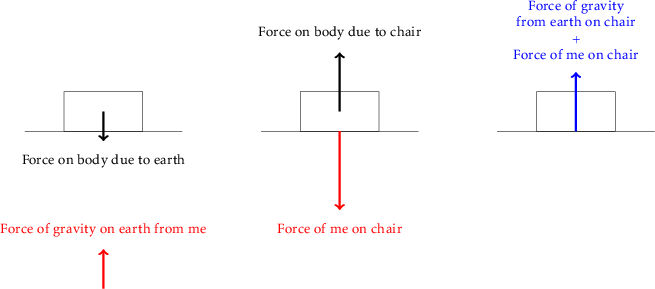Hopefully our time with free-body diagrams separates the action-reaction pairs for us all into the appropriate diagrams: whichever diagram would could the force as external to itself gets it.
The chair in the above diagram pushes upwards with 588 N on a giant Tickle-me Elmo. What force does he exert on the chair?Since Newton’s Third Law tells us the forces have to be equal in magnitude but opposite in direction, so Elmo exerts a force of -588 N on the chair.

What happens when we run into a wall? Or punch one? The wall hits us right back, which is why it hurts, and why it’s generally not a good idea to run into walls.

Action and reaction forces don’t cancel each other out, and each object could still accelerate from the action-reaction interaction, depending on the sum of forces on each object. For example, two astronauts next to each other in space could both accelerate, in opposite directions, from one pushing the other.

### Common Mistakes

Action-reaction force pairs are never the force of gravity countered by a normal force. The magnitudes may be the same, but that doesn’t make them an action-reaction pair.

Only label forces acting on an object in its free-body diagram, and not the ones it exerts itself, such as its gravitation pull on Earth or its push on whatever surface it’s sitting on.

If it seems that a problem doesn’t have enough information to go on, start labeling everything given, and listing all equations relevant to the information available. For example, we can find acceleration from velocity and time, or distance and a pair of times. As long as acceleration’s constant, we can use any of the projectile equations.

### Brain Snack

Galileo and Isaac Newton had more than their scientific interests in common: they were both Renaissance men. They sought new knowledge, building on the work of the Ancients.